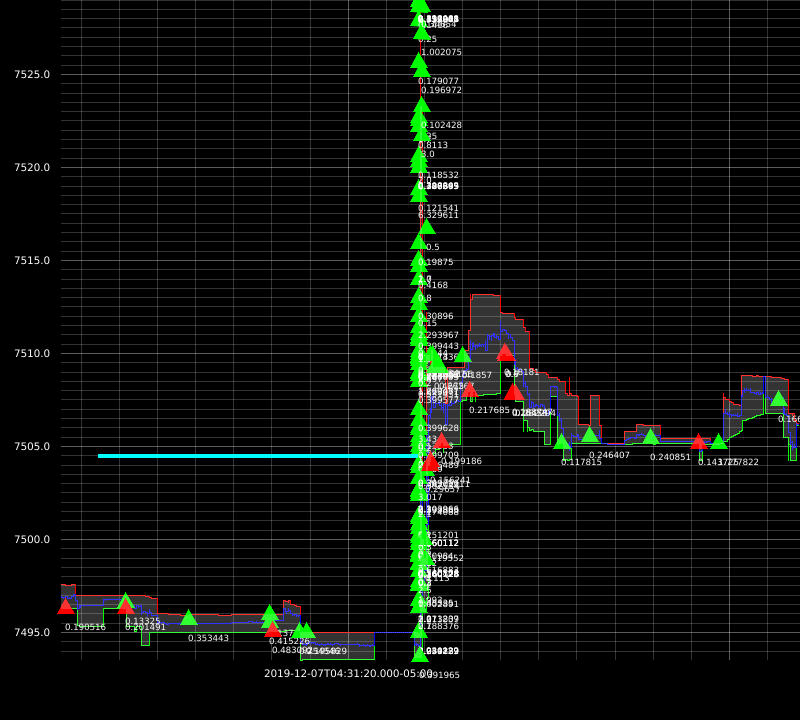Recently, I worked on market microstructure simulation to backtest a HFT strategy. In this post, I will show you how to build a simple but effective simulator. Hope you find it helpful.

# Goal

In the past, I worked on strategies whose inputs are based on OHLC candles, but developing microstructure based strategies requires microstructure level simulation: post LOs to historical orderbook states, replay subsequent events and get execution info.

# The first simulator: Poisson Process

Avellaneda-Stoikov’s model of orderflow intensity is used to estimate probability of execution. It doesn’t simulate microstructure but works for backtests. The model is based on market dynamics and requires 2 parameters that can be estimated using tick data.

The idea is: arrival rate of MOs matching posted depth $$\delta$$ at time $$t$$ iis modeled by a Poisson Process with intensity

\begin{align} \lambda(t, \delta) &= \alpha(t) P(\Delta p > \delta) \\ &= \alpha(t) e^{-\mu \delta} \end{align}

where $$\alpha(t)$$ is the current fill probability at best price, the arrival rate of MO at time $$t$$ and $$P(\Delta p > \delta)$$ is probability that the size of the MO would be greater than the size of all LOs of price less than $$\delta$$ combined. To see how it is derived you need to look at bottom left of page 220.

Note: the parameters $$\alpha(t)$$ and $$\mu$$ are estimated for each of 10 minute segments in backtest period.

The binary $$x \in [fill, no fill]$$ is sampled from a Bernoulli distribution: $$B(\lambda(t, \delta)\Delta)$$ where $$\Delta$$ is the strategy-dependent maximum time interval before cancellation.

It’s very fast to compute - it takes ~20 seconds to backtest a month’s data using 32 threads on a Threadripper 1950X. However, some LOs had questionable fills in some scenarios I visualized. So I decided to build a real orderbook microstructure simulator.

# The second simulator: SimBook

I implemented a market simulator called SimBook based on a flowchart from Robert Almgren’s slides which was very helpful(thank you!).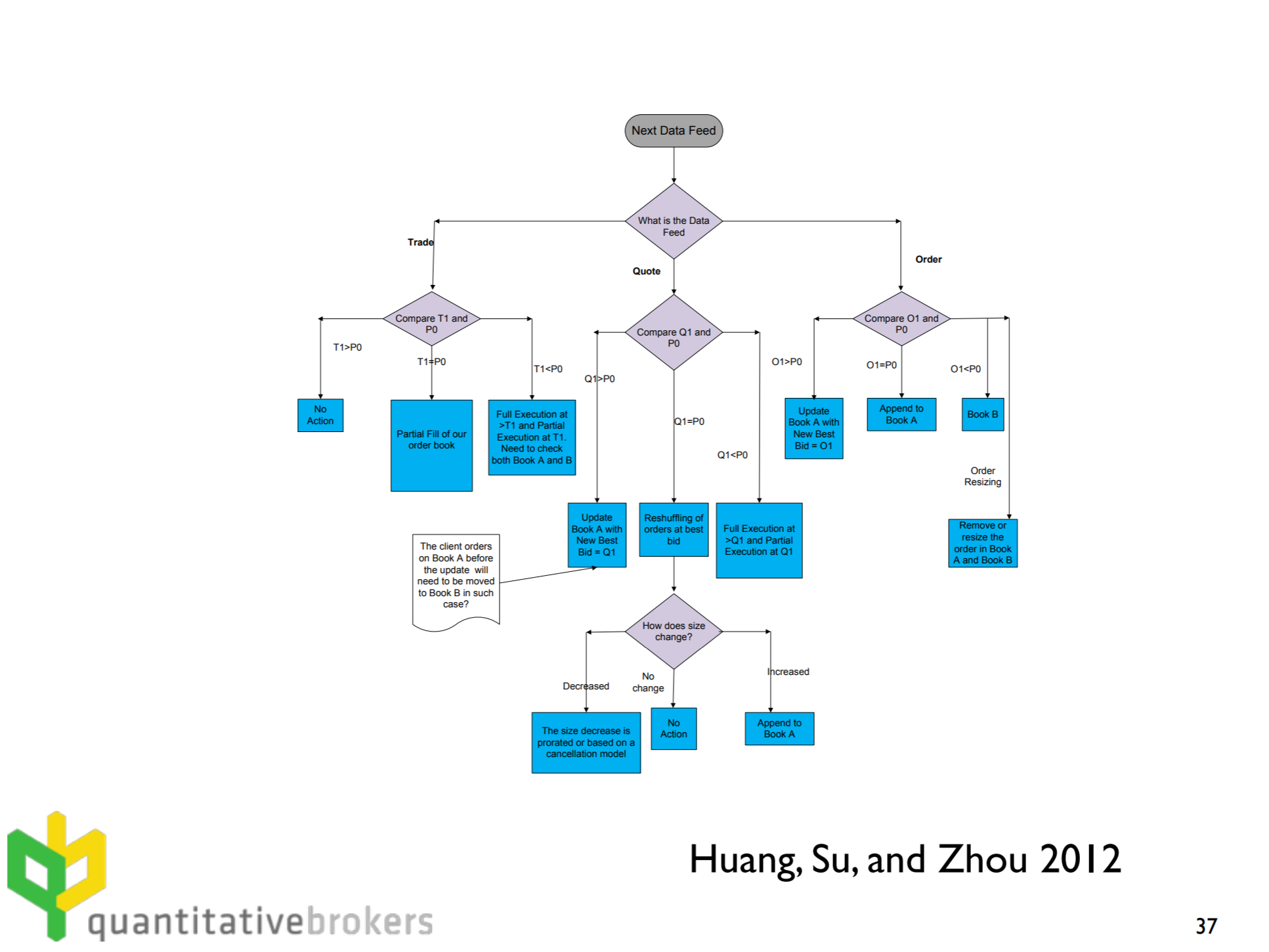The idea is to build an orderbook matching engine that follows a set of pessimistic exchange matching rules.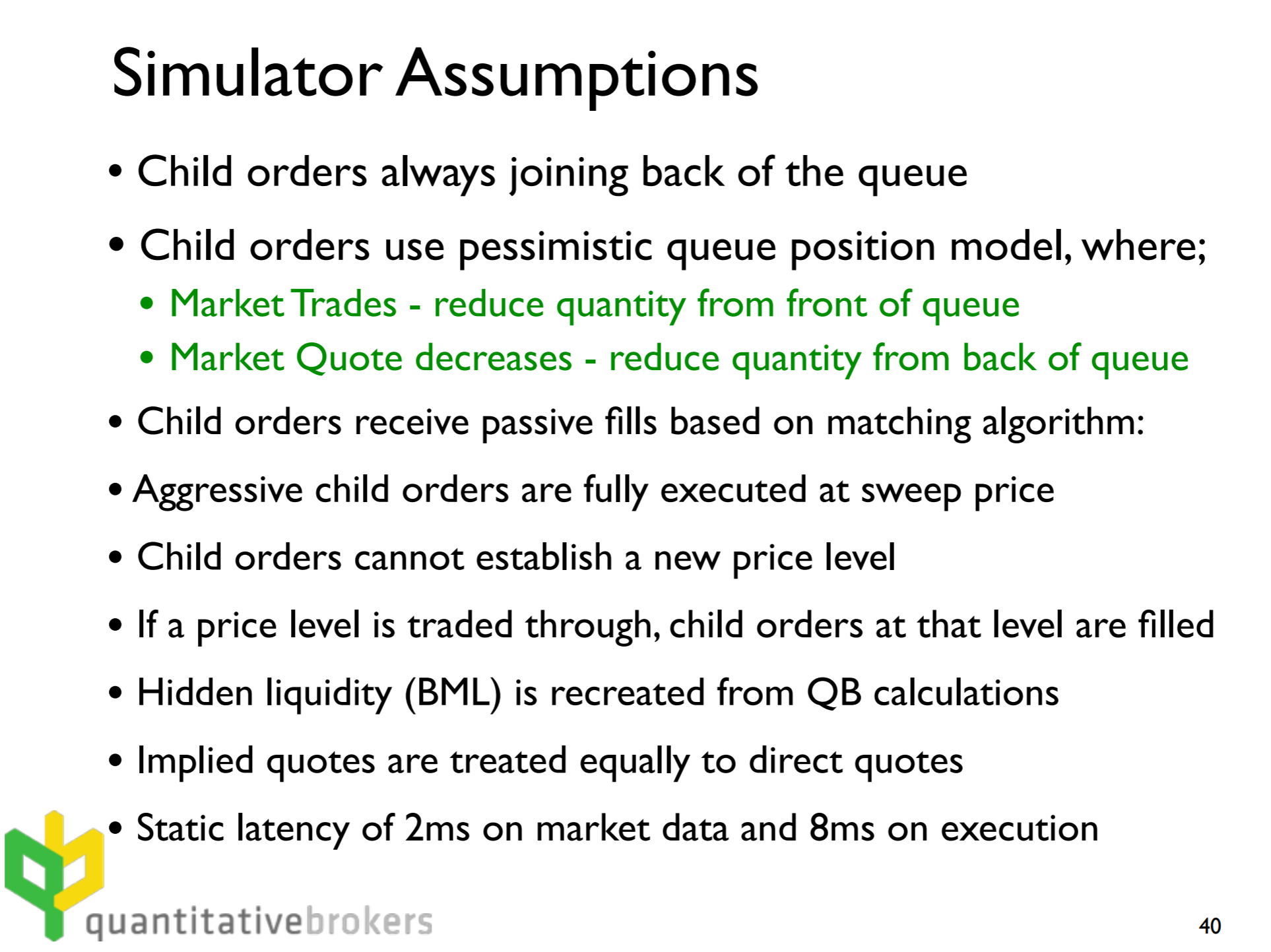Since I couldn’t find the original paper “Combining historical data with a market simulator for testing algorithmic trading” (presumably because it was a term paper for a course hence never published), it was hard to understand some of the rationale behind these design decisions but it was mostly self-explanatory.

For my implementation, I relaxed some assumptions but they don’t affect realism too much. For example, simulated orders are allowed to establish new price levels as long as they are worse than bba. I also implemented some heuristics for maximum order size and latency restrictions which makes it slightly more realistic.

I was able to achieve great results with this approach. Here are some example executions from my backtests: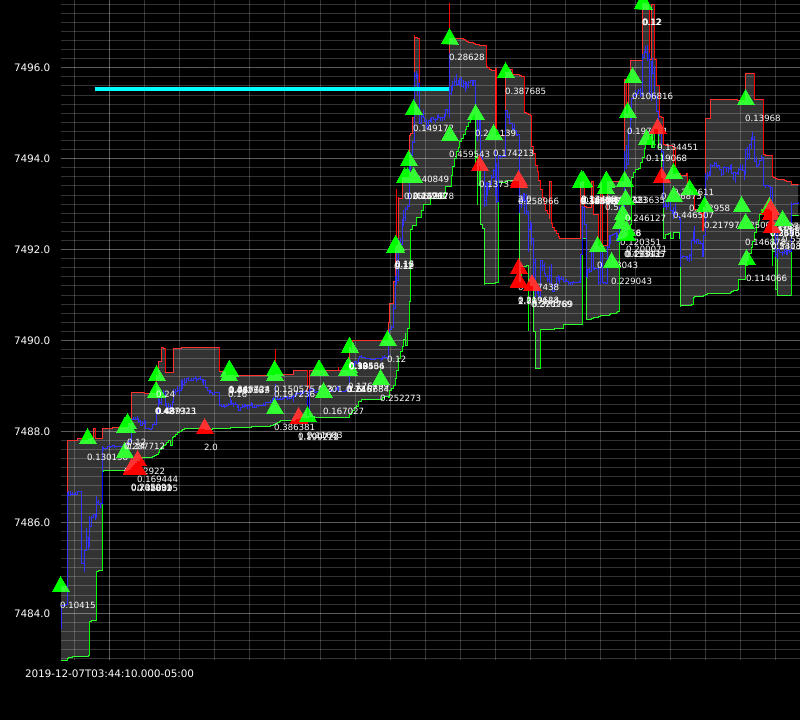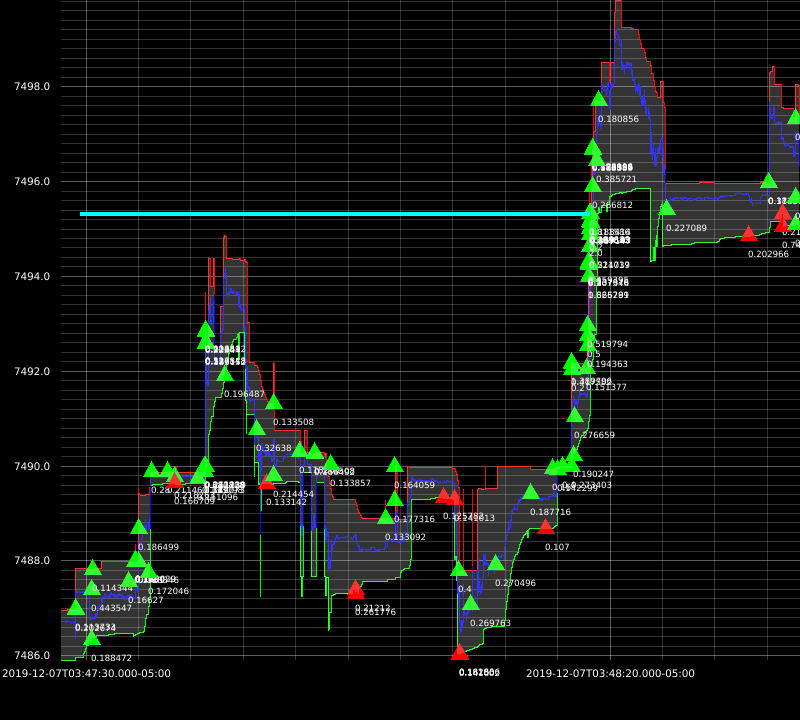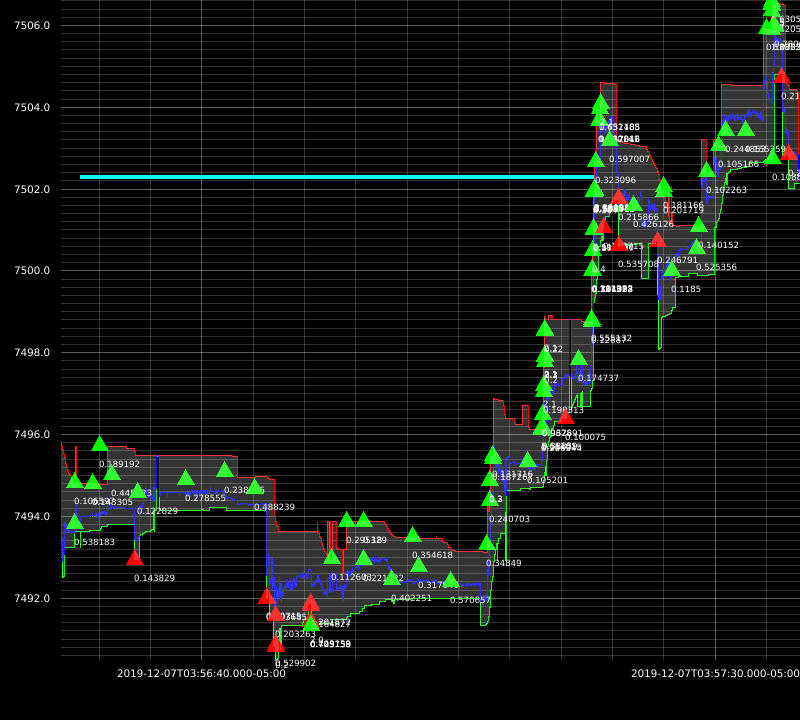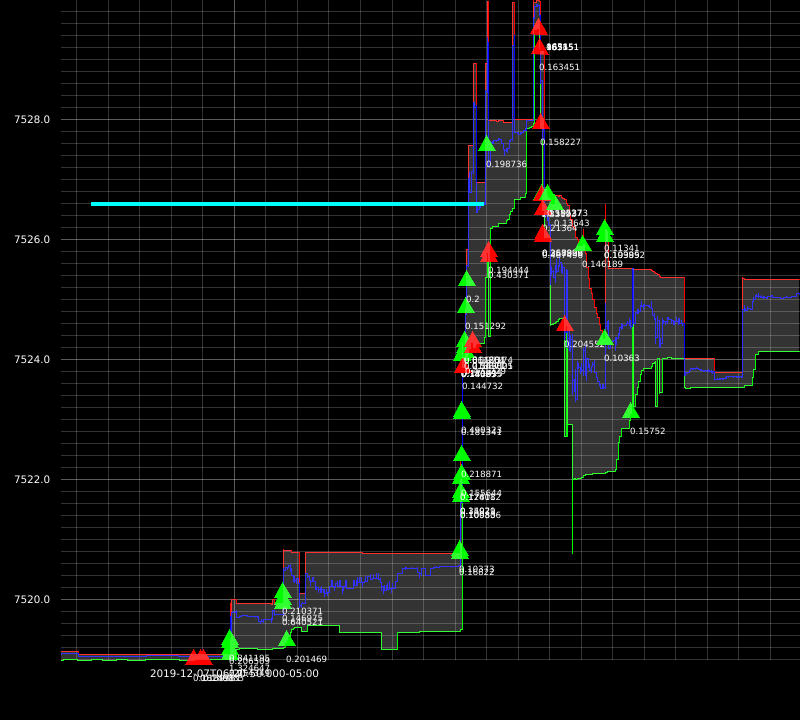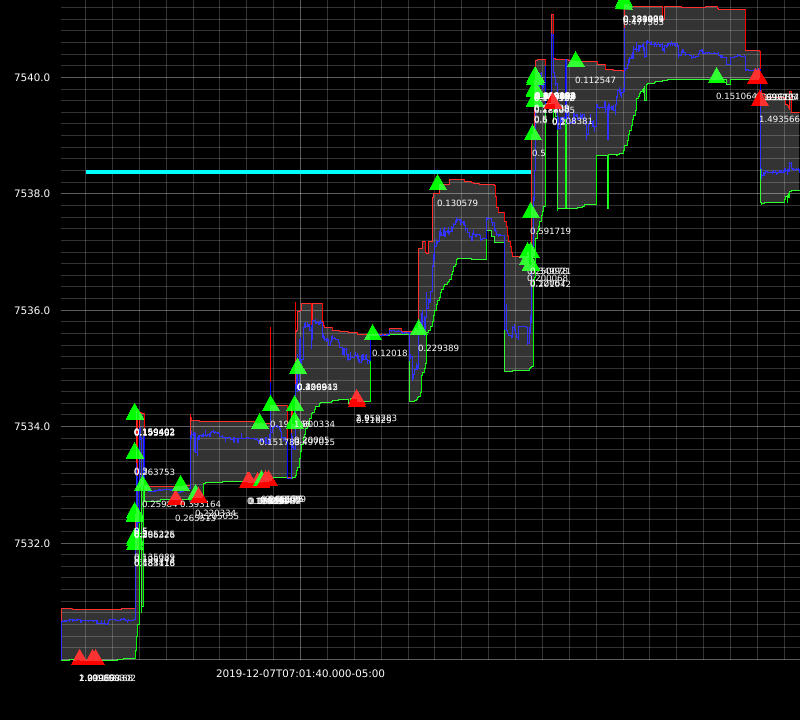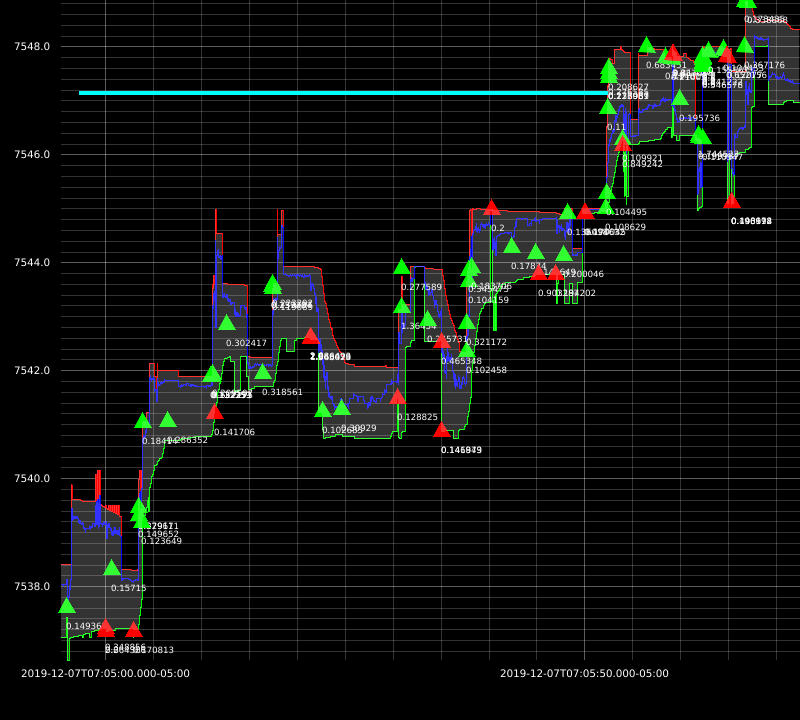The downside of this approach is: market impact not being taken into account. At this time, my strategies are dumb so I don’t need more sophisticated simulators just yet.

# The third simulation: Queue-Reactive Model

This one I did not implement but explored in depth. I got inspired to develop a signal that uses Kalman filter to estimate the implicit spread. I will explain this in a separate blog post.